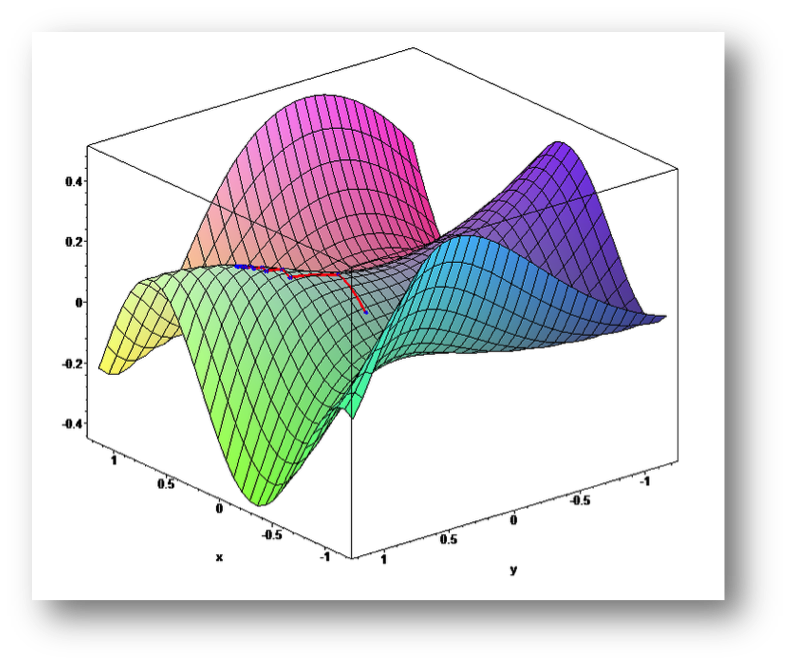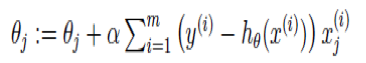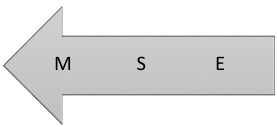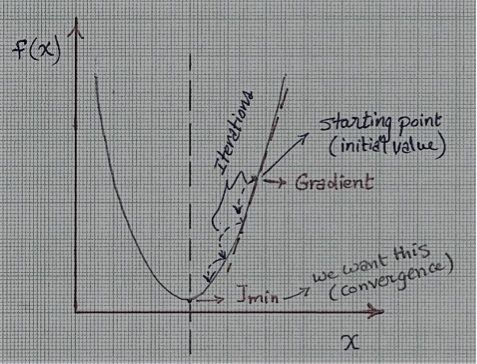Machine Learning vs. Statistical Modeling

There are lots of differences between traditional statistical modeling and machine learning. Machine learning depends on computer algorithms, few estimates, and small to large datasets to produce predictions of high accuracy.

Statistical modeling, on the other hand, depends on human talents, mathematical equations, and many assumptions to predict the “best estimate.”### How much training data do I need to develop my model?

The amount of training data needed to build a comprehensive machine learning model is highly debated. The type of data determines the number of features. The greater the number of features, the greater the amount of data needed (e.g., bioinformatics and genetics models will need more training data than real estate models). The golden rule is that the amount of data should be 10 times the number of features. However, this is also debatable because in some cases, gathering enough data is the real issue (imagine building a model for tsunamis).

Another factor is the choice of evaluation model. Evaluation models may use the same dataset to train and test or may follow the train/test split methodology.

John Snow Labs has cleaned and curated over 1,800 datasets across 18 areas of human health and well-being. We also have a team of healthcare research specialists at your service. You can check out the John Snow Labs data library here.

Our top five datasets by number of data points:

1. 51,350,000
2. 24,525,000
3. 18,275,000
4. 14,375,000
5. 11,675,000

KDnuggets™ has a good blog addressing how much data is needed for more complex models. Practically speaking, the domain experience and plotting a learning curve are the best ways to decide whether you have enough data. These will expose the training progress and test errors while you increase the training dataset size.

### The Gradient Descent (J) and Linear Regression

You need to develop a model to be able to predict the outputs. Assume that you have a function H(X). This function is defined by a set of parameters or coefficients Ɵ0, Ɵ1, Ɵ2, Ɵ3…etc.) and can be represented as:

H0 (X) = Ɵ0 + Ɵ1 X

This equation is a linear equation, meaning that the solution set can be represented by a straight line. The closer the value of H(X) to the value of Y in the coordinates (X, Y) of the training data, the more precise the data model will be. In other words, you need the minimum difference between the estimator (training dataset) and the estimated value (prediction) to determine that you have a good model. This can only be achieved by choosing values for Theta (Ɵ) or for the parameters that can achieve this minimum difference.

You must use a formula called the “Cost Function [J(Ɵn)]” to calculate the error (use the Mean Squared Error [MSE] function). The MSE function will measure the difference between the training dataset and the predicted values.(Cost Function)Your next goal should be to minimize the value of this function (to minimize the error). This can be accomplished by using an algorithm called “Gradient Descent Algorithm.” Gradient Descent is a simple algorithm used for optimization. Use it whenever parameters (coefficients) cannot be calculated analytically (using linear algebra).

Gradient Descent uses the following function iteratively by assigning an initial value (weight) to the coefficients and then changing these values iteratively proportional to the negative of the function gradient until it reaches convergence. Convergence can be achieved by looking for minor changes in error iteration-to-iteration (where the gradient is close to zero value).This equation will be repeated for every (j) until convergence is reached.

Hint: (α) in the equation represents the learning rate. You can initially consider it a value representing how fast you can reach the minimum or simply how much time this process may take.

The efficiency of Gradient Descent with very large training datasets has been questioned. Regardless, machine learning experts can scale Gradient Descent to very large datasets.

There two main types of Gradient Descent are Batch Gradient Descent and Stochastic Gradient Descent. Batch Gradient Descent can be used with linear and logistic regression, but it is somewhat slow and not suitable for very large datasets. Stochastic Gradient Descent is much faster and more suitable for large training datasets.

Not only is obtaining training data a challenge, but other challenges include determining how much data you need, cleaning and curating the data, and choosing the best optimization algorithm and evaluation methodology. If you are a data scientist, this will require your valuable time to achieve sound results. Tasks like data curation and choice of methodology can be outsourced to a consultant. The bigger challenge is finding the right experts to assist you. John Snow Labs is the organization you need to keep your (J) at the minima and significantly mitigate the risks of your research project.### About Mohamed Tharwat